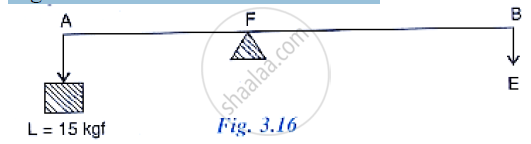Share
Notifications

View all notifications
Books Shortlist
Your shortlist is empty

# Fig 3.16 Below Shows a Lever in Use. (A) to Which Class of Lever Does It Belong? (B) If Ab = 1m, Af = 0.4 M, Find Its Mechanical Advantage, (C) Calculate the Value of E. - ICSE Class 10 - Physics

Login
Create free account

Forgot password?

#### Question

Fig 3.16 below shows a lever in use.(a) To which class of lever does it belong?
(b) If AB = 1m, AF = 0.4 m, find its mechanical advantage,
(c) calculate the value of E.

#### Solution

(a) This is a class I lever.
(b) Given AB=1m, AF=0.4m and BF=0.6 m
Mechanical advantage M.A = 𝐵𝐹𝐴𝐹= 0.60.4=1.5
(c) Load = 15kfg
Effort = "𝐿𝑜𝑎𝑑"/"𝑀.𝐴" = 15/1.5 = 10 𝑘𝑔𝑓

Is there an error in this question or solution?

#### APPEARS IN

Solution Fig 3.16 Below Shows a Lever in Use. (A) to Which Class of Lever Does It Belong? (B) If Ab = 1m, Af = 0.4 M, Find Its Mechanical Advantage, (C) Calculate the Value of E. Concept: Concept of Lever.
S